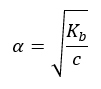# Degree of ionization

Equilibrium: Degree of ionization

Degree of ionization refers to the strength of an acid or base to form ions in solution. While strong acids/base completely ionize in solution, weak acids/base ionize partially. Likewise, in a weak acid dynamic equilibrium is established between compounds where the chemical equilibrium constant can be expressed.

Recall that strong acids dissociate completely into ions in water.

HA(g or l) + H2O(l)H3O+ (aq) + A(aq) Kc>>1

Weak acids dissociate minimally into ions in water

HA(aq) + H2O(l) ↔ H3O+ (aq) + A(aq) Kc<<1

In this equation, Kc stands for the equillibrium constant which can be used to define the acid dissociation constant, Ka.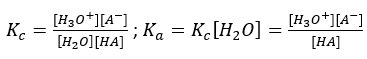Stronger acids have higher [H3O+] making larger Kawhile weaker acids have lower [H3O+] making smaller Ka.

In both cases, [H3O+] from the auto-ionization of water is negligible but a weak acid has a small Ka so we can assume that [HA]dissocis very small. Likewise,

[HA]eq = [HA]init – [HA]dissoc ≈ [HA]init

As the initial concentration of a weak acid decreases, the percent dissociation of the acid increases.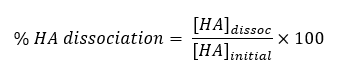Similarly for bases, the expression can be illustrated as:

A(aq) + H2O(l) ↔ OH(aq) + HA+(aq) Kc<<1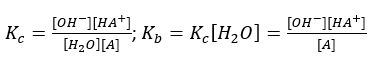Here, Kb is called the base ionization constant.

Degree of ionization is expressed as α. For strong electrolytes, α≈1. Examples include HCl, HNO3, NaCl, KOH etc. As for weak electrolytes α<<1. Examples include HCN, CH3COOH, H2O. Strong electrolyte with a common ion decreases the degree of ionization.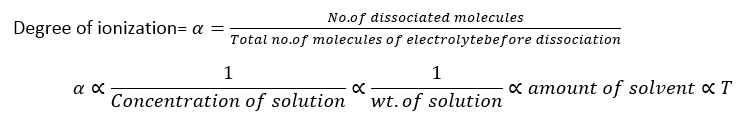The degree of ionization decreases with increase in the concentration of the solution. With more solvent, more ions are readily available for ionization which increases the degree of ionization. Also, as the temperature increases the kinetic energy of the solution increases and more molecules collide with each other to facilitate solute-solvent interaction. Hence, as the temperature increases, the degree of ionization also increases.Figure : Effect of added common ion. In this case, acetate ion is added to acetic acid to analyze its degree of dissociation. As the amount of acetate ion increase, the degree of dissociation decreases.

Consider a weak acid that partially dissociates in an aqueous solution. Here,if we know Ka of the acid, then we can find initial concentrations of conjugate acid and base. We need to make assumptions about the amount of acid-dissociating relative to its initial concentration and proceed step wise through changes in the system.

Assume c and an as the initial moles of CH3COOH and H2O respectively and α as the degree of dissociation. The concentration of water does not change noticeably with the reaction since it is present in excess.

 moles CH3COOH(aq) +H2O(l)CH3COO–(aq) +H3O+(aq) Initial c a 0 0 Change – α a α α Equilibrium c(1-α) a cα cα

The equilibrium constant can be written as: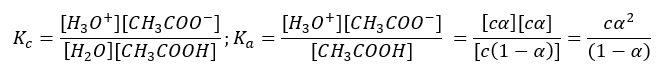Since the acid is weak the value of (1-α) is negligible.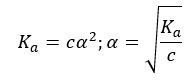This means that acid dissociation constant can be used to calculate the degree of ionization. Similarly, base dissociation constant is also related to the degree of dissociation where,